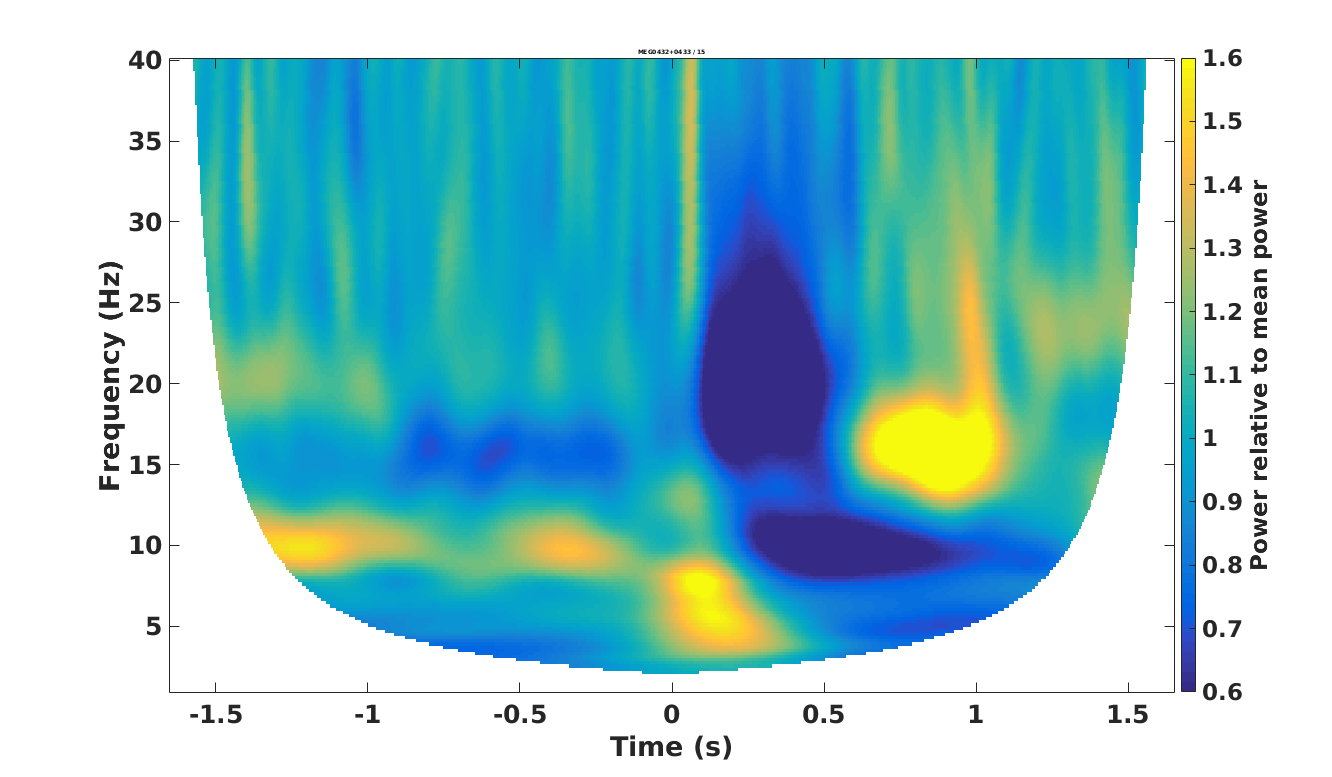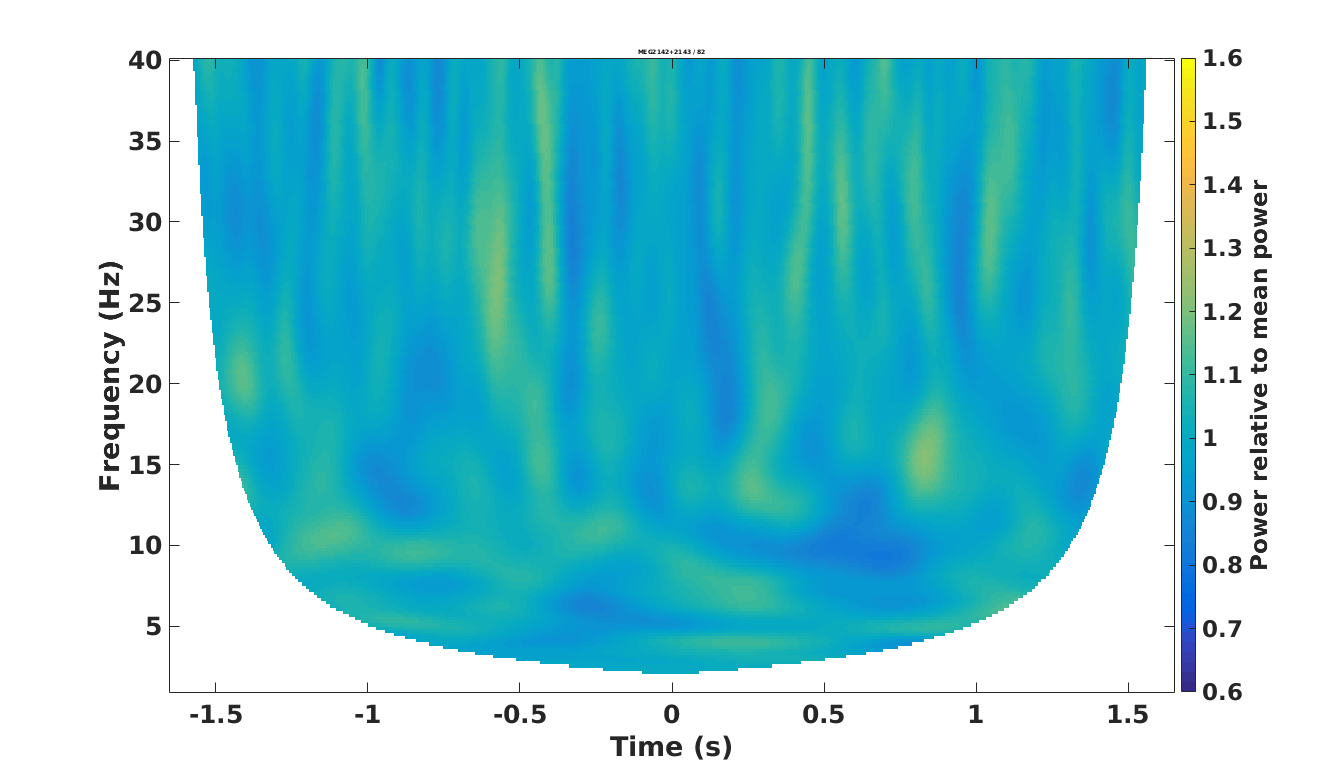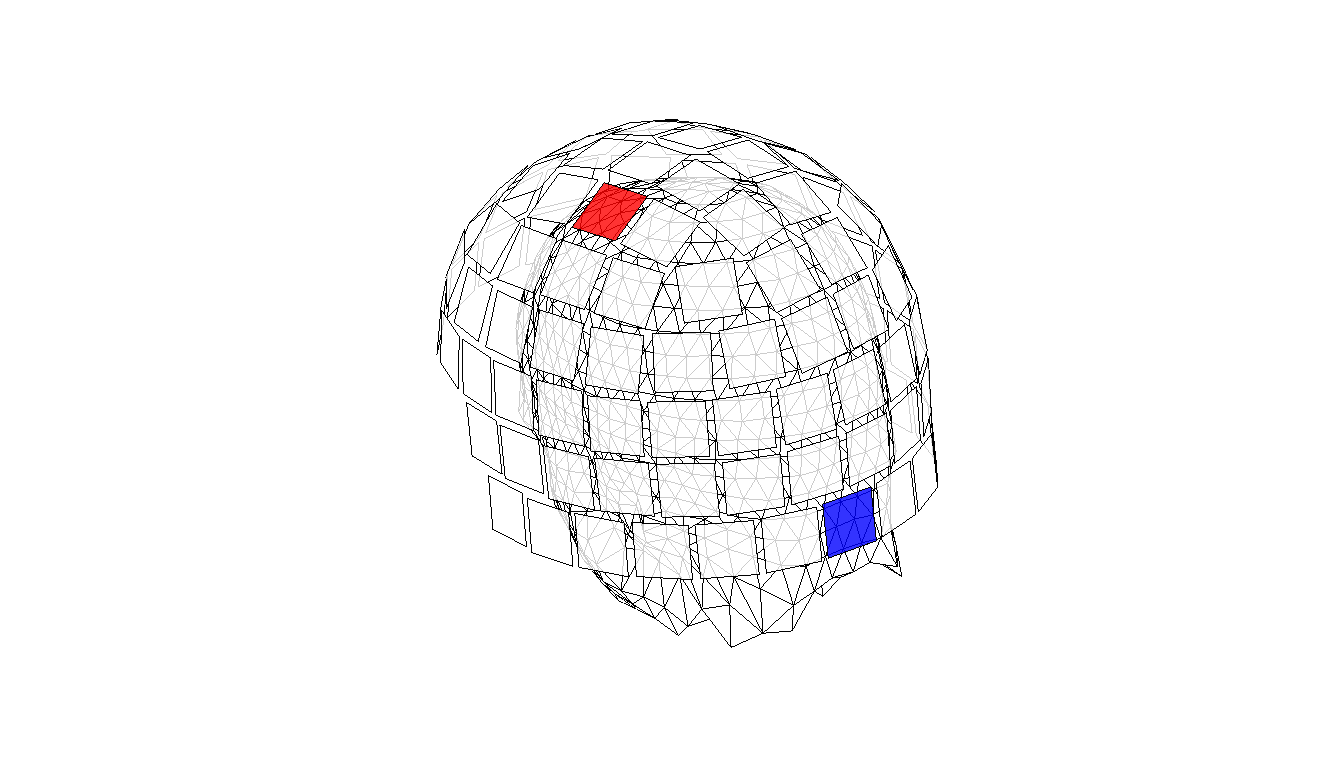(leave them as is, but do evaluate the sections)
The paths simply serve as an example of how you can set up your analysis structure, which is especially useful if you have more than one subject

### Set up general paths

Change these to appropriate paths for your operating system and setup
(leave them as is, but do evaluate the section)

``````%% paths

ft_defaults

raw_meg_path = '/archive/20067_workshop_source_reconstruction/MEG/';
meg_path = '/home/share/workshop_source_reconstruction/data/MEG/';
mri_path = '/home/share/workshop_source_reconstruction/data/MRI/';
set(0, 'DefaultAxesFontWeight', 'bold', 'defaultaxesfontsize', 36, 'defaultlinelinewidth', 2);
``````

### Set up subject specific paths

Make subject and recording specific paths (the cell array “subjects_and_dates” can be expanded)

``````%% subjects and dates

subjects_and_dates = ...
{
'NatMEG_0177/170424/'
};

output_path = fullfile(meg_path, subjects_and_dates{1});

events = [1 2 4 8 16]; %% right little, right ring, right middle, right index, right thumb
``````

### Load files necessary for the beamforming

1. We are loading the TFRs to locate interesting targets for beamformer source reconstruction
4. We are loading the preprocessed data (baseline_data) on which we will do the actual source reconstruction
``````%% go to relevant path and load data

cd(output_path)
disp Done``````

### Set channels

Here you can set which channels you want to base the source reconstruction on
You only need to change to one of the three options specified

``````%% set channels

if strcmp(channels, 'eeg')
sensor_type = 'eeg';
else
sensor_type = 'meg';
end``````

### Identify interesting features

Plot all the channels in the data
Notice (at least) three features of interest

1. The so-called beta rebound from about 700 to 1000 msec at around 16 Hz
2. The so-called mu desynchronization from about 300 to 800 msec at around 10 Hz
3. The so-called high-beta desynchronization from about 200 to 500 msec at around 22 Hz
``````%% identify interesting features in the data

% pick an event
event_index = 1; %% can be anything from 1-5

cfg = [];
cfg.layout = 'neuromag306cmb.lay'; %% this is combined gradiometers, you can also choose <'neuromag306mag.lay'> or <'neuromag306eeg1005_natmeg.lay'
cfg.baselinetype = 'relative'; %% absolute power is not terribly meaningful; therefore we use 'relative' to look at increases and decreases in power relative to the overall power level
cfg.baseline = [-Inf Inf]; %% from min to max
cfg.colorbar = 'yes'; %% show the interpretation of the colours
% cfg.zlim = [0.6 1.6]; %% play around with this parameter to familiarize yourself with these plots

ft_multiplotTFR(cfg, combined_tfrs{event_index});``````

### Single channel pair plot, MEG0432+0433### Single channel pair plot, MEG2142+2143### Visualizing channels

``````%% channels that we'll plot throughout

colours = ones(306, 3);
tactile_channel = 'MEG0432';
occipital_channel = 'MEG2142';
tactile_channel_index = find(strcmp(baseline_data.label, tactile_channel));
occipital_channel_index = find(strcmp(baseline_data.label, occipital_channel));

colours(tactile_channel_index, :)   = [1 0 0]; %% make red
colours(tactile_channel_index + 1, :)   = [1 0 0]; %% make red
colours(occipital_channel_index, :) = [0 0 1]; %% make blue
colours(occipital_channel_index + 1, :) = [0 0 1]; %% make blue

figure('units', 'normalized', 'outerposition', [0 0 1 1]);
hold on
ft_plot_sens(sensors, 'facecolor', colours, 'facealpha', 0.8);

view([-45 25])``````

### Showing where the channels are (we will use these throughout)### The beta rebound

We will here focus on reconstructing the activity underlying the beta rebound

1. We crop the data to find the time period of interest (690 to 970 msec)
2. We define a baseline period of similar duration. We will compared the time period of interest against this, since the power estimates that the beamformer results in are not terribly informative
3. We also making a combination of the time period of interest and the baseline. (Its use will become apparent later)
``````%% time window of interest Beta Rebound

beta_toi = [0.690 0.970];
baseline_toi = [-0.500 -0.220];
n_events = length(events);

tois_rebound = cell(1, n_events);
tois_baseline  = cell(1, n_events);

for event_index = 1:n_events

event = events(event_index);

cfg = [];
cfg.toilim = beta_toi;
cfg.trials = baseline_data.trialinfo == event;

tois_rebound{event_index} = ft_redefinetrial(cfg, baseline_data);

cfg.toilim = baseline_toi;

tois_baseline{event_index} = ft_redefinetrial(cfg, baseline_data);

end

% combined data

tois_combined = cell(1, n_events);

for event_index = 1:n_events

cfg = [];

tois_combined{event_index} = ft_appenddata(cfg, tois_rebound{event_index}, tois_baseline{event_index});

end``````

### Example plot timelocked

The main lesson here is that there is no timelocked activity

``````%% example plot tois

event_index = 1;
channel_of_interest = 'MEG0431';
toi_rebound  = tois_rebound{event_index};
toi_baseline = tois_baseline{event_index};
channel_index = strcmp(channel_of_interest, toi_rebound.label);
n_samples = length(toi_rebound.time{1});
n_trials = length(toi_rebound.trial);

figure('units', 'normalized', 'outerposition', [0 0 1 1]);
hold on
for n_trial = 1:n_trials
plot(1:n_samples, toi_rebound.trial{n_trial}(channel_index, :), 'r')
end
xlabel('Sample no');
ylabel('Magnetic Field Strength (T)');
title(channel_of_interest)
for n_trial = 1:n_trials
plot(1:n_samples, toi_baseline.trial{n_trial}(channel_index, :), 'b')
end``````